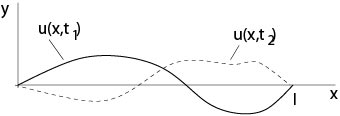$$\newcommand{\id}{\mathrm{id}}$$ $$\newcommand{\Span}{\mathrm{span}}$$ $$\newcommand{\kernel}{\mathrm{null}\,}$$ $$\newcommand{\range}{\mathrm{range}\,}$$ $$\newcommand{\RealPart}{\mathrm{Re}}$$ $$\newcommand{\ImaginaryPart}{\mathrm{Im}}$$ $$\newcommand{\Argument}{\mathrm{Arg}}$$ $$\newcommand{\norm}{\| #1 \|}$$ $$\newcommand{\inner}{\langle #1, #2 \rangle}$$ $$\newcommand{\Span}{\mathrm{span}}$$

# 1.1: Examples

$$\newcommand{\vecs}{\overset { \rightharpoonup} {\mathbf{#1}} }$$ $$\newcommand{\vecd}{\overset{-\!-\!\rightharpoonup}{\vphantom{a}\smash {#1}}}$$$$\newcommand{\id}{\mathrm{id}}$$ $$\newcommand{\Span}{\mathrm{span}}$$ $$\newcommand{\kernel}{\mathrm{null}\,}$$ $$\newcommand{\range}{\mathrm{range}\,}$$ $$\newcommand{\RealPart}{\mathrm{Re}}$$ $$\newcommand{\ImaginaryPart}{\mathrm{Im}}$$ $$\newcommand{\Argument}{\mathrm{Arg}}$$ $$\newcommand{\norm}{\| #1 \|}$$ $$\newcommand{\inner}{\langle #1, #2 \rangle}$$ $$\newcommand{\Span}{\mathrm{span}}$$ $$\newcommand{\id}{\mathrm{id}}$$ $$\newcommand{\Span}{\mathrm{span}}$$ $$\newcommand{\kernel}{\mathrm{null}\,}$$ $$\newcommand{\range}{\mathrm{range}\,}$$ $$\newcommand{\RealPart}{\mathrm{Re}}$$ $$\newcommand{\ImaginaryPart}{\mathrm{Im}}$$ $$\newcommand{\Argument}{\mathrm{Arg}}$$ $$\newcommand{\norm}{\| #1 \|}$$ $$\newcommand{\inner}{\langle #1, #2 \rangle}$$ $$\newcommand{\Span}{\mathrm{span}}$$

Example 1.1.1:

$$u_y=0$$, where $$u=u(x,y)$$. All functions $$u=w(x)$$ are solutions.

Example 1.1.2:

$$u_x=u_y$$, where $$u=u(x,y)$$. A change of coordinates transforms this equation into an equation of the first example. Set $$\xi=x+y,\ \eta=x-y$$, then
$$u(x,y)=u\left(\frac{\xi+\eta}{2},\frac{\xi-\eta}{2}\right)=:v(\xi,\eta).$$
Assume $$u\in C^1$$, then
$$v_\eta=\frac{1}{2}(u_x-u_y).$$
If $$u_x=u_y$$, then $$v_\eta=0$$ and vice versa, thus $$v=w(\xi)$$ are solutions for arbitrary $$C^1$$-functions $$w(\xi)$$. Consequently, we have a large class of solutions of the original partial differential equation: $$u=w(x+y)$$ with an arbitrary $$C^1$$-function $$w$$.

Example 1.1.3:

A necessary and sufficient condition such that for given $$C^1$$-functions $$M,\ N$$ the integral
$$\int_{P_0}^{P_1}\ M(x,y)dx+N(x,y)dy$$
is independent of the curve which connects the points $$P_0$$ with $$P_1$$ in a simply connected domain $$\Omega\subset\mathbb{R}^2$$ is that the partial differential equation (condition of integrability)
$$M_y=N_x$$
in $$\Omega$$.Figure 1.1.1: Independence of the path

This is one equation for two functions. A large class of solutions is given by $$M=\Phi_x,\ N=\Phi_y$$, where $$\Phi(x,y)$$ is an arbitrary $$C^2$$-function. It follows from Gauss theorem that these are all $$C^1$$-solutions of the above differential equation.

Example 1.1.4: Method of an integrating multiplier for an ordinary

differential

equation

Consider the ordinary differential equation
$$M(x,y)dx+N(x,y)dy=0$$
for given $$C^1$$-functions $$M,\ N$$. Then we seek a $$C^1$$-function $$\mu(x,y)$$ such that $$\mu Mdx+\mu Ndy$$ is a total differential, i. e., that $$(\mu M)_y=(\mu N)_x$$ is satisfied. This is a linear partial differential equation of first order for $$\mu$$:
$$M\mu_y-N\mu_x=\mu (N_x-M_y).$$

Example 1.1.5:

Two $$C^1$$-functions $$u(x,y)$$ and $$v(x,y)$$ are said to be functionally dependent if
$$\det\left(\begin{array}{cc}u_x&u_y\\v_x&v_y\end{array}\right)=0,$$
which is a linear partial differential equation of first order for $$u$$ if $$v$$ is a given $$C^1$$-function. A large class of solutions is given by
$$u=H(v(x,y)),$$
where $$H$$ is an arbitrary $$C^1$$-function.

Example 1.1.6: Cauchy-Riemann equations

Set $$f(z)=u(x,y)+iv(x,y)$$, where $$z=x+iy$$ and $$u,\ v$$ are given $$C^1(\Omega)$$-functions. Here $$\Omega$$ is a domain in $$\mathbb{R}^2$$. If the function $$f(z)$$ is differentiable with respect to the complex variable $$z$$ then $$u,\ v$$ satisfy the Cauchy-Riemann equations
$$u_x=v_y,\ \ u_y=-v_x.$$
It is known from the theory of functions of one complex variable that the real part $$u$$ and the imaginary part $$v$$ of a differentiable function $$f(z)$$ are solutions of the Laplace equation
$$\triangle u=0,\ \ \triangle v=0,$$
where $$\triangle u= u_{xx}+u_{yy}$$.

Example 1.1.7: Newton Potential

The Newton potential
$$u=\frac{1}{\sqrt{x^2+y^2+z^2}}$$
is a solution of the Laplace equation in $$\mathbb{R}^3\setminus{(0,0,0)}$$, that is, of
$$u_{xx}+u_{yy}+u_{zz}=0.$$

Example 1.1.8: Heat equation

Let $$u(x,t)$$ be the temperature of a point $$x\in\Omega$$ at time $$t$$, where $$\Omega\subset\mathbb{R}^3$$ is a domain. Then $$u(x,t)$$ satisfies in $$\Omega\times[0,\infty)$$ the heat equation
$$u_t=k\triangle u,$$
where $$\triangle u= u_{x_1x_1}+u_{x_2x_2}+u_{x_3x_3}$$ and $$k$$ is a positive constant. The condition
$$u(x,0)=u_0(x),\ \ x\in \Omega,$$
where $$u_0(x)$$ is given, is an initial condition associated to the above heat equation. The condition
$$u(x,t)=h(x,t),\ \ x\in \partial\Omega,\ t\ge0,$$
where $$h(x,t)$$ is given, is a boundary condition for the heat equation.

If $$h(x,t)=g(x)$$, that is, $$h$$ is independent of $$t$$, then one expects that the solution $$u(x,t)$$ tends to a function $$v(x)$$ if $$t\to\infty$$. Moreover, it turns out that $$v$$ is the solution of the boundary value problem for the Laplace equation
\begin{eqnarray*}
\triangle v&=&0\ \ \mbox{in}\ \Omega\\
v&=&g(x)\ \ \mbox{on}\ \partial\Omega.
\end{eqnarray*}

Example 1.1.9: Wave equationFigure 1.1.2: Oscillating string

The wave equation
$$u_{tt}=c^2\triangle u,$$
where $$u=u(x,t)$$, $$c$$ is a positive constant, describes oscillations of membranes or of three dimensional domains, for example. In the one-dimensional case
$$u_{tt}=c^2 u_{xx}$$
describes oscillations of a string.

Associated initial conditions are
$$u(x,0)=u_0(x),\ \ u_t(x,0)=u_1(x),$$
where $$u_0,\ u_1$$ are given functions. Thus the initial position and the initial velocity are prescribed.

If the string is finite one describes additionally boundary conditions, for example
$$u(0,t)=0,\ \ u(l,t)=0\ \ \mbox{for all}\ t\ge 0.$$

## Contributors

• Integrated by Justin Marshall.